# Chapter 3 Assignment Statement An assignment statement gives

• Slides: 16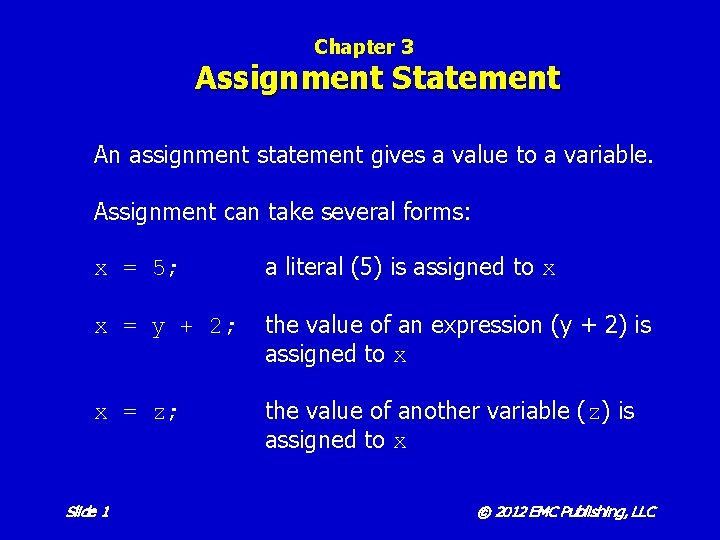Chapter 3 Assignment Statement An assignment statement gives a value to a variable. Assignment can take several forms: x = 5; a literal (5) is assigned to x x = y + 2; the value of an expression (y + 2) is assigned to x x = z; the value of another variable (z) is assigned to x Slide 1 © 2012 EMC Publishing, LLC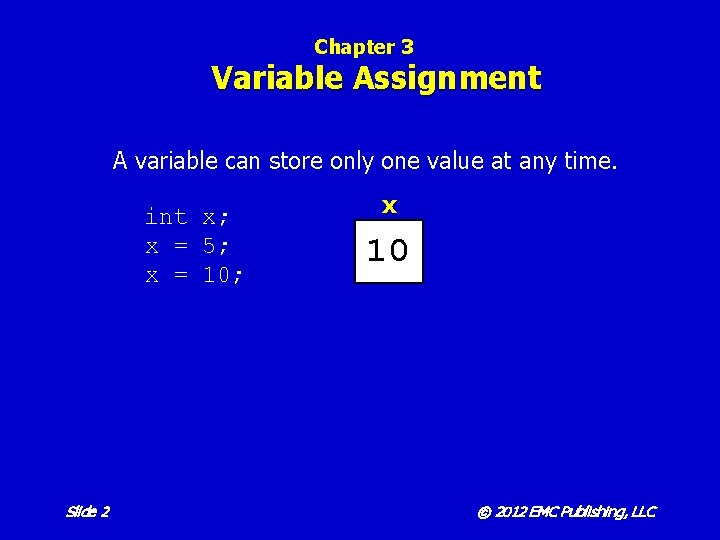Chapter 3 Variable Assignment A variable can store only one value at any time. int x; x = 5; x = 10; Slide 2 x 10 5 © 2012 EMC Publishing, LLCChapter 3 Primitive Data Types Type int double char boolean Slide 3 Storage Required 4 bytes 8 bytes 2 bytes 1 bit © 2012 EMC Publishing, LLC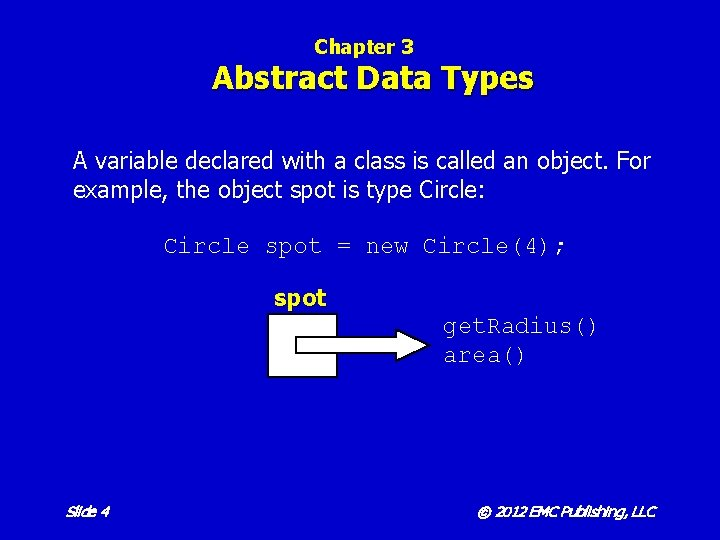Chapter 3 Abstract Data Types A variable declared with a class is called an object. For example, the object spot is type Circle: Circle spot = new Circle(4); spot get. Radius() area() Slide 4 © 2012 EMC Publishing, LLC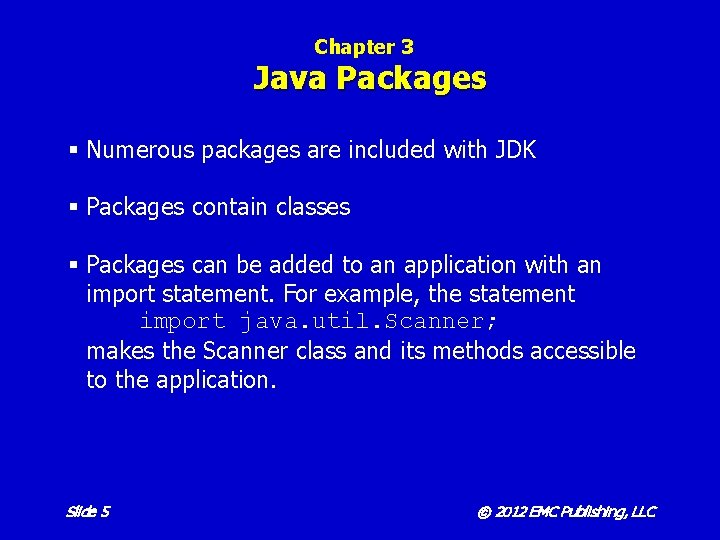Chapter 3 Java Packages § Numerous packages are included with JDK § Packages contain classes § Packages can be added to an application with an import statement. For example, the statement import java. util. Scanner; makes the Scanner class and its methods accessible to the application. Slide 5 © 2012 EMC Publishing, LLCChapter 3 The Scanner Class § Part of the java. util package § A Scanner object processes text and numbers from the input stream § Methods include: next() next. Line() next. Int() next. Double() next. Boolean() close() Slide 6 © 2012 EMC Publishing, LLCChapter 3 Integer Division Integer division (/) is performed when both operands are integers. Only the integer portion of the quotient is returned: Slide 7 © 2012 EMC Publishing, LLC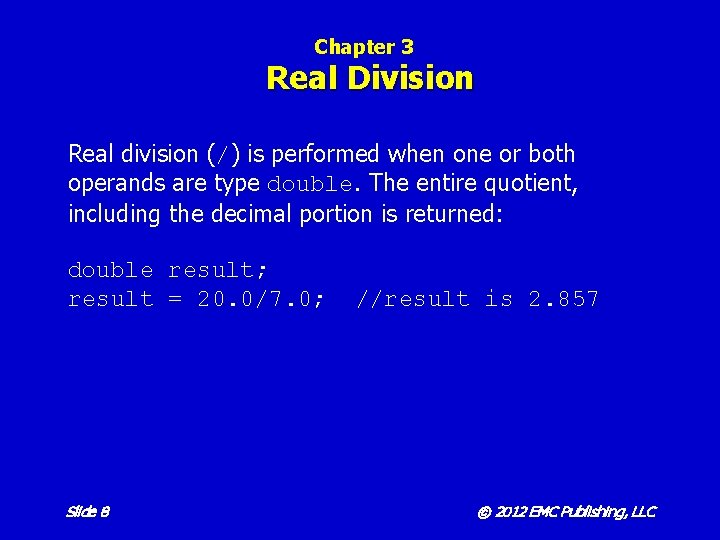Chapter 3 Real Division Real division (/) is performed when one or both operands are type double. The entire quotient, including the decimal portion is returned: double result; result = 20. 0/7. 0; Slide 8 //result is 2. 857 © 2012 EMC Publishing, LLC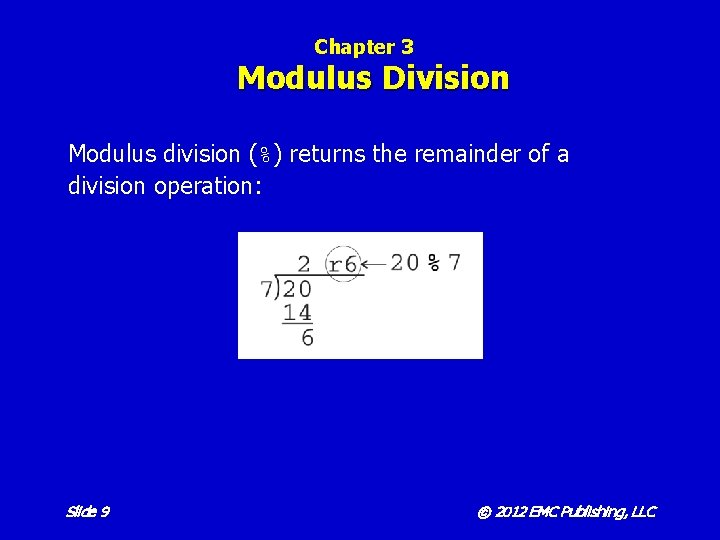Chapter 3 Modulus Division Modulus division (%) returns the remainder of a division operation: Slide 9 © 2012 EMC Publishing, LLCChapter 3 Operator Precedence Operators in Java have the following precedence: 1. multiplication and division 2. addition and subtraction Operators of the same precedence are evaluated in order from left to right. For example, multiplication is performed first, then division, and finally addition: 5 + 6 * 4 / 2 = 17 Slide 10 © 2012 EMC Publishing, LLCChapter 3 Changing the Order of Operations The order in which operators are evaluated can be changed by using parentheses. For example, addition is performed first, then multiplication, and finally division: (5 + 6) * 4 / 2 = 22 Slide 11 © 2012 EMC Publishing, LLCChapter 3 Type Casting converts a number of one type to a number of a different, but compatible type. Type casting is used to: 1. make the operand types in an expression match. For example, whole. Num = (int)y * 2 2. truncate the decimal portion of a double. For example, whole. Num = (int)z 3. change the way in which a division (/) operation will be performed. For example, real. Division = (double)a / (double)b Slide 12 © 2012 EMC Publishing, LLC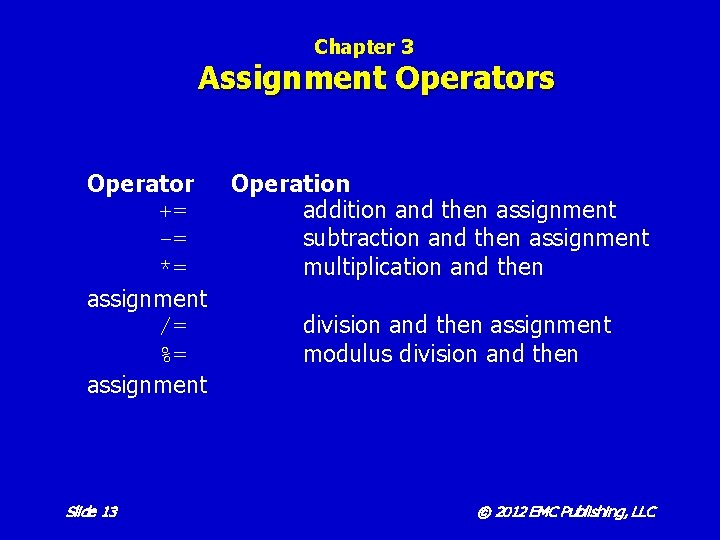Chapter 3 Assignment Operators Operator Operation += addition and then assignment -= subtraction and then assignment *= multiplication and then assignment /= division and then assignment %= modulus division and then assignment Slide 13 © 2012 EMC Publishing, LLC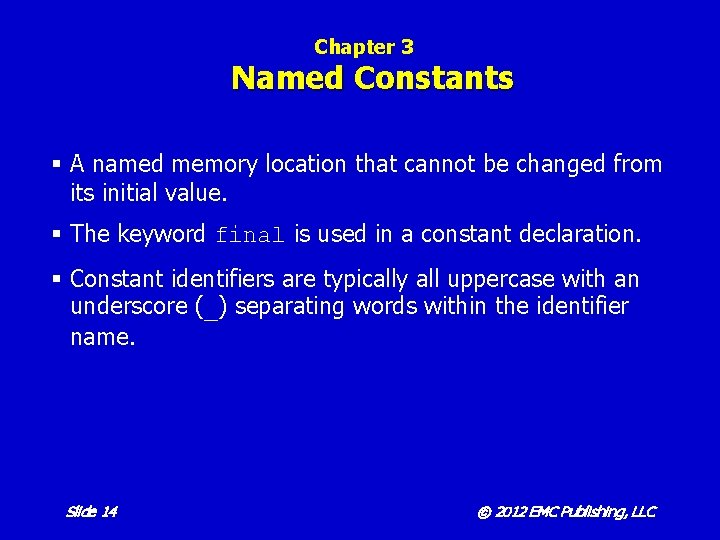Chapter 3 Named Constants § A named memory location that cannot be changed from its initial value. § The keyword final is used in a constant declaration. § Constant identifiers are typically all uppercase with an underscore (_) separating words within the identifier name. Slide 14 © 2012 EMC Publishing, LLC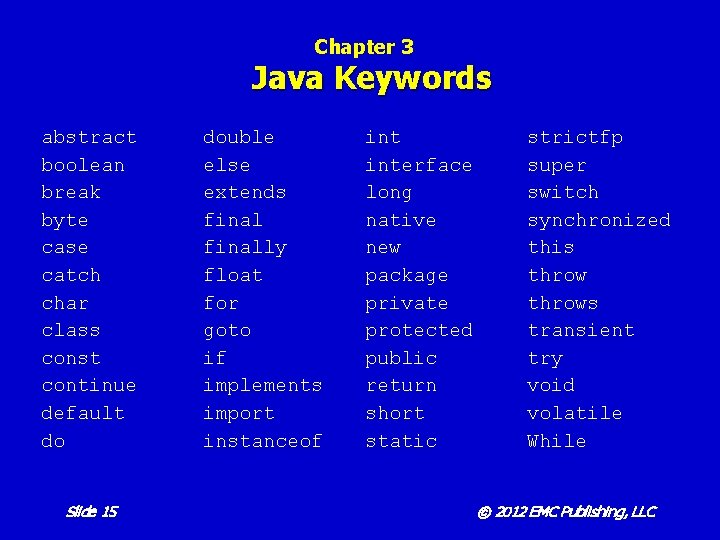Chapter 3 Java Keywords abstract boolean break byte case catch char class const continue default do Slide 15 double else extends finally float for goto if implements import instanceof interface long native new package private protected public return short static strictfp super switch synchronized this throws transient try void volatile While © 2012 EMC Publishing, LLCChapter 3 Programming Errors § Syntax errors violate the rules of Java. § Logic errors, also called semantic errors, occur in statements that are syntactically correct, but produce undesired or unexpected results. § Run-time errors, also called exceptions, halt program execution at the statement that cannot be executed. One type of exception is called Input. Mismatch. Exception. Slide 16 © 2012 EMC Publishing, LLC